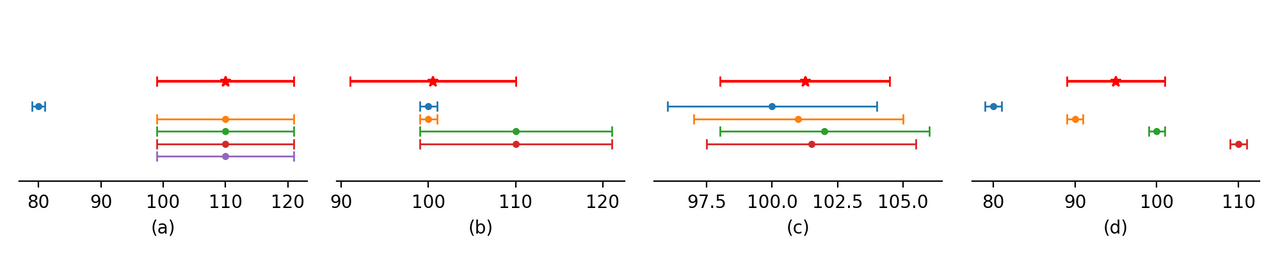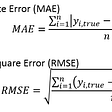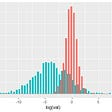# Pyth Price and Confidence Aggregation

At Pyth, we’ve been working on a new algorithm for combining the price feeds of publishers into a single aggregate price feed, and we’d like to share it with the community for feedback before releasing it.

As background, Pyth is an oracle that publishes an aggregate price and confidence interval for each product on every Solana slot. The Pyth program computes this price on-chain by aggregating the prices and confidence intervals submitted by individual publishers. For example, one publisher may say that the price of BTC/USD is \$52000 ± 10 and another that it is \$53000 ± 20, and Pyth may combine these two prices into an aggregate price of \$52500 ± 500. This post proposes a new aggregation algorithm for this final step.

Goals

We would like Pyth’s aggregation algorithm to have three properties. First, it should be robust to manipulation — both accidental and intentional — by publishers. If most publishers are submitting a price of \$100 and one publisher submits a price of \$80, the aggregate price should remain near \$100 and not be overly influenced by the single outlying price. This property ensures that the Pyth price remains accurate even if a small number of publishers submit a price that is far from the market. This scenario is depicted as graph (a) in the figure below.Scenarios for the aggregation procedure. The lower thin bars represent the prices and confidence intervals of each publisher, and the bold red bar represents where we intuitively would like the aggregate price and confidence to be.

Second, the aggregate price should appropriately weight data sources with different levels of accuracy. Pyth allows publishers to submit a confidence interval because they have varying levels of accuracy in observing the price of a product. For example, US equity exchanges have different levels of liquidity, and less liquid exchanges have wider bid/offer spreads than more liquid ones. This property can result in situations where one exchange reports a price of \$101 +- 1, and another reports \$110 +- 10. In these cases, we would like the aggregate price to be closer to \$101 than \$110. This scenario is depicted as graph (b) in the figure.

Finally, the aggregate confidence interval should reflect the variation between publishers’ prices. In reality, there is no single price for any given product. At any given time, every product trades at a slightly different price at various venues around the world. Furthermore, a trader will obtain a different price if they immediately buy or sell the product. We would like Pyth’s confidence interval to reflect these variations in prices across venues. Graphs (c) and (d) in the figure depict two different cases where there are price variations across exchanges.

Aggregation Algorithm

We designed an aggregation algorithm to obtain these three properties. The first step of the algorithm computes the aggregate price by giving each publisher three votes — one vote at their price and one vote at each of their price plus and minus their confidence interval — then taking the median of the votes. The second step computes the distance from the aggregate price to the 25th and 75th percentiles of the votes, then selects the larger of the two as the aggregate confidence interval.

This simple algorithm has some surprising properties: the procedure for computing the aggregate price is in fact a generalization of the ordinary median. Most people understand the median as the middle value in the data set, that is, the 50th percentile. However, the median is also the value R that minimizes the objective function ∑ᵢ |R — pᵢ| where pᵢ is the price of each publisher. This function penalizes R based on its distance from the publisher’s price pᵢ. Pyth’s algorithm computes the aggregate price R that minimizes ⅓Σᵢ|R — pᵢ| + ⅔Σᵢ max(|R — pᵢ| — cᵢ, 0), where cᵢ is the publisher’s confidence interval. This objective combines the ordinary median objective with a second term that only assigns a penalty to R if it lies outside the publisher’s confidence interval. This objective function encourages the aggregate price R to not only be close to the publishers’ prices, but also lie within their confidence intervals. (There is a simple proof of the equivalence between the voting scheme and minimizing this objective function that we will leave to the reader.)

We can visualize both objective functions to understand the difference between them:

The left is objective function of the ordinary median applied to a price pᵢ= 0, which is simply |R|. The right is Pyth’s objective function ⅓|R| + ⅔max(|R| — cᵢ, 0) depicted for various choices of confidence interval cᵢ. The width of the confidence interval determines the location of the kink in the curve — as the interval widens, the kink moves further from 0. The region between the two kinks has reduced penalty relative to the median.

This generalization of the median has the first two properties we wanted. It is robust to outliers because the ordinary median is robust to outliers. (It’s easy to see that the median is robust — the 50th percentile of your dataset is unchanged no matter what the value of the 99th percentile is.) Furthermore, its confidence interval adjustment accounts for the accuracy of different data sources.

We can visualize this objective function in the scenarios from above as follows:

The plots above depict the objective function as a red line. The optimum of this objective function is the red star, which is the location of the aggregate price. Each publisher’s contribution to the objective is depicted as a grey dashed line. Thus, the sum of the grey dashed functions is the red function. The leftmost graph shows the robustness of the objective function, and the 2nd graph shows how publishers with tighter confidence intervals exert greater influence over the location of the aggregate price. In both the third and fourth graphs, the objective function gives identical results to the ordinary median.

The second step of the aggregation algorithm computes a confidence interval around the aggregate price. Pyth’s aggregate confidence interval can be viewed as a generalization of the interquartile range, a standard measure of the dispersion of a data set. The interquartile range is typically defined as the distance between the 25th and 75th percentiles. We modified this definition to take the maximum of the distance between the aggregate price and the 25th or 75th percentile in order to produce a confidence interval that is symmetric around the aggregate price. Furthermore, instead of using the interquartile range of the prices, Pyth uses the interquartile range of the publishers’ votes. This modification accounts for both the width of publishers’ confidence intervals and any dispersion between publishers’ aggregate prices.

Putting everything together, the following figure shows the behavior of the complete algorithm on our four scenarios:

The red star depicts the aggregate price, as computed using the median algorithm, and the corresponding bold red line depicts the aggregate confidence interval. The grey circles represent the 25th and 75th percentiles of the votes — the further one of these from the aggregate price determines the aggregate confidence interval’s width. The other elements of the plot are identical to those from the plot above. The third graph shows that the aggregate confidence interval accounts for publishers’ confidence intervals, and the fourth graph shows that it accounts for price dispersion between publishers.

Theoretical Analysis

In addition to producing intuitively reasonable results, this algorithm has nice theoretical properties. The aggregate price is guaranteed to be equal to the ordinary median in two limiting scenarios:

1. The publishers publish the same price, but with confidence intervals of varying widths. In this case, the votes will be sorted such that the bottom 33 percent consists of the price minus the confidence interval, and the top 33 percent consists of the price plus the confidence interval. The median of the votes is equal to the median of the 33rd-66th percentiles, which is the ordinary median of the publishers’ prices. This scenario is depicted in the 3rd graph from the left above.
2. The publishers publish distinct prices with non-overlapping confidence intervals. In this case, all votes of a single publisher will be adjacent in the sorted list and we can treat them as a single vote. Therefore, Pyth’s aggregate price reduces to the median of the publisher’s prices. This scenario is depicted in the 4th graph from the left above.

Furthermore, even if we are not in one of these scenarios, we can prove that the aggregate price will never be too far from the ordinary median in rank terms. More specifically, the aggregate price will always lie within the 25th-75th percentile of the publisher’s prices. This robustness property means that as long as fewer than 25% of the publishers are erroneous, they cannot completely determine the location of the aggregate price. (Note that the ordinary median would give you a stronger guarantee, 50% instead of 25%. The difference between these bounds is due to the case where some publishers have a wide confidence interval and other publishers are moving their prices around inside that interval. We actually want the tighter publishers to have more influence in this case!)

Note that all of the results here generalize to the case where the publishers have varying weights in the computation. We’re working on a staking system for publishers that incentivizes them to provide accurate data, and in that system, each publisher will have a varying amount of stake. All of the results also hold for stake weights if we simply replace the % of publishers with the % of stake controlled.

Conclusion

So there it is — a complete introduction to Pyth’s new aggregation algorithm. The algorithm itself is simple. Each publisher submits a price pᵢ and confidence cᵢ that we use to produce three votes for the publisher, pᵢcᵢ, pᵢ, pᵢ+ cᵢ. The median of all the votes is the aggregate price, and the 25th — 75th percentile, symmetrized around the aggregate price, is the aggregate confidence interval. This algorithm is a confidence-adjusted variant of the ordinary median that yields intuitive results and also has solid theoretical properties. We’d love to hear your feedback, so feel free to reach out to us on Discord with questions or comments.

--

--

--

## More from Pyth Network

Smarter data for smarter contracts. Pyth is designed to bring real-world data on-chain on a sub-second timescale.

Love podcasts or audiobooks? Learn on the go with our new app.

## Combining Technical Analysis With K-Means## Life Insurance Risk Prediction using Machine Learning Algorithms- Part II: Algorithms and Results## In Search of the Next Best Data Story## The Worst Misconception in Statistics## To the Aspiring Data Scientists## Data Science project life cycle## Three new grants awarded to strengthen data-driven vaccine delivery## Some fun facts about statistical studies## Pyth Network

Smarter data for smarter contracts. Pyth is designed to bring real-world data on-chain on a sub-second timescale.

## New Pyth Data Provider: Raydium## Drift Protocol Launches: Limit Orders## Saber Launches UXD with USDC on Solana!## Introducing Accrue Finance — a new Solana product that maximizes your APY